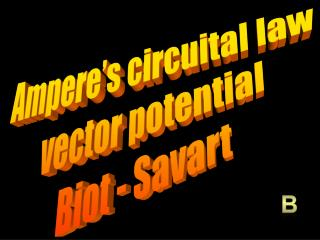Download PresentationAmpere’s circuital law vector potential Biot - Savart

# Ampere’s circuital law vector potential Biot - Savart - PowerPoint PPT PresentationDownload Presentation## Ampere’s circuital law vector potential Biot - Savart

- - - - - - - - - - - - - - - - - - - - - - - - - - - E N D - - - - - - - - - - - - - - - - - - - - - - - - - - -
##### Presentation Transcript

1. Ampere’s circuital law vector potential Biot - Savart b

2. * Turn the compass needle so it is approximately parallel to the wire. * Close the switch to send the current through the wire for about 5-10 seconds. * The compass will align itself with the magnetic field.

3. B a I Ampere’s circuital lawright hand rule

4. positive charge out of screen B

5. B a I

6. B a r B a I 0  r < a

7. B a r B a I a  r

8. concentric hollow cylinders

9. B 0- 0 a b c r

10. + L solenoid superposition

11. Thomson Lord Kelvin 1824-1907

12. vector potential A we know that • B = 0 we know that • [ x vector] = 0 we can now specify the vector let vector be A such that B =  x A William Thomson shows that Neumann's electromagnetic potential A is in fact the vector potential from which may be obtained via B =  x A.

13. vector potential A B =  x A we also know  x B = µoj  x  x A  = • A) -  -   A = - µoj is similar to Poisson’s equation but we have to solve three PDE’s A and j are in the same direction!!

14. j(r’) A(r) r r’

15. z dz’ 2 L z’ R A r I

16. z dz’ 2 L z’ R A r I after the integration

17. Biot 1774-1862 Savart 1791-1841 Biot-Savart law

18. Slide through the integral! Biot-Savart law

19. Biot-Savart law

20. 0 Biot-Savart law

21. Biot-Savart law

22. j(r’) B(r) + r’ r ur’ - r Biot-Savart law

23. z dz’ 2 L z’ R B r I

24. z dz’ 2 L z’ R B r I

25. magnetic dipole

26. B B B js A summary Three techniques to find B 1] Ampere’s circuital law - lots of symmetry 2] find vector potential A, then B =  x A 3] Biot - Savart law